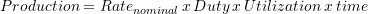### A Brief Guide to Engineering Financial Calculations: The Income Statement & Contribution Margin

Key Assumptions

In this course, unless otherwise stated, the Income Statement is considered to be a financial statement of earnings before interest and taxes, which is of particular interest to operations managers. Cost accounting allocates costs according to categories that relate to whether the cost is directly related to production or indirectly related overhead. How indirect costs are allocated to a product can give a clear (or distorted) picture of the overall cost of a product.

There is an alternative accounting method called activity-based accounting. This method links each activity that the organization does to specific cost drivers (e.g., machining, transportation, procurement, engineering,…), and tries to minimize the amount of overhead charges. This method of accounting can be more accurate, but it requires care in budgeting and cost tracking. Activity-based accounting is not covered in this course.

Revenue is the money that comes into the company through sales of its goods or services.

Costs directly related to production are generally included in the category of Cost of Goods Sold (COGS).

Costs that are not directly related to production are generally listed under the category of Sales General & Administrative (SG&A). Depreciation is an element of the expenses that are included within SG&A. The depreciation is a non-cash expense that represents the loss in asset value during the reporting period. The other charges against income that are non-cash charges are:

• Allowances for future costs.
• Allowances for reclamation.

These would also go into cash from operations. We do not usually consider these allowances in most of our work.

Other Income includes one-time revenues and expenses such as writedowns. In some companies, the interest on the long-term debt is also included within Other Income.

Contribution margin represents the value that has been created above what is necessary to cover direct costs; that value can cover the indirect costs and, with Other Income, (hopefully) create profit (also called earnings). In this part of the course we are dealing with how an operation generates earnings, which may be for a part of a company. For this reason, we consider the earnings before paying any interest expenses for borrowed money, or taxes. This is an advantage when considering the CM of different product lines.

When a new opportunity for a company appears, and the indirect costs have already been covered, then the profit of that incremental is very high. This is because there are little to no indirect costs associated with the extra revenue. That means that such work can be discounted to some extent, and still make a profit (provided that all of the direct costs are covered, of course).

Calculations

Net Revenue = Gross Revenue – (Bad debt, warranty, and allowances)

Contribution Margin = Net Revenue – COGS

Contribution Margin (%) = (Net Revenue – COGS) / Gross Revenue

Operating Income = Contribution Margin – SG&A (including Depreciation). (Operating Income is usually calculated before considering any taxes.)

Net Income = Operating Income + Other Income.

Net Income is also called Profit or Earnings. If Net Income is negative it is a Loss. Remember, the definition of Net Income used here is the same as Earnings before Interest or taxes (EBIT). Later in the course we will consider Net Income for an entire company, including costs of financing (interest) and income taxes.

Quick checks in the Income Statement to assess whether there is enough cash being generated to run the operation are: Cash Flow from Operations, and Net Cash Flow.

Cash Flow from Operations = Operating Income + Depreciation. (typically)

= Contribution Margin – SG&A (excluding depreciation)

Net Cash Flow = Net Income + Depreciation + Writedowns. (typically)

Net Cash Flow is also called Total Cash Flow. Here we have assumed that the only Other Income is writedowns.

Cash break-even is when operating income (with any any non-cash expenses such as depreciation having been added back in) is zero. Book break-even is when operating income is zero without having to add depreciation back in (that is, when the non-cash expense is subtracted). Cash break-even thus occurs at a lower operating income than book break-even. Cash breakeven means that you aren’t making a profit, but at least you are not losing money in the short term. Book breakeven means that not only can you cover your costs, but also you replace assets if necessary. Cash breakeven point can be simply calculated from:

Simple cash breakeven point = SG&A / (Net Revenue – COGS)

Production

Production over an interval of time is the product of: the nominal rate of production, the duty (which is the fraction of the nominal production rate that is actually achieved, the utilization (the fraction of the time that production actually occurs), and the time interval:This formula assumes that the nominal production rate is a good representation of the average production rate. In many cases this is a reasonable assumption; but in some industries the production rate varies considerably, due to the batch nature of production or other influences.